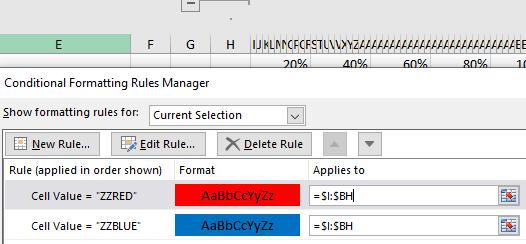### Formulas in XTT

Direct editing of formulas from the code is prohibited, as this approach is prone to errors and is not very informative.
In addition, the internal representation of formulas in Excel xlsx (absolute) and Excel xml (relative) does not allow using a uniform approach if formulas were filled from the ABAP side. Instead, there are a few (hopefully simple) rules for editing formulas in MS Excel itself.

### Copying data

New lines (and cells) are added by copying the original line in the template. Formulas are copied along with cell data, borders, and other formatting.

To prevent the formulas from “shifting” during copying cells, you can use several methods:

The most native and reliable way for Excel is to calculate distance from the current cell where we write the formula, you could use =OFFSET() in conjunction with =INDIRECT() for creating relative formulas.

those, to get the sum of the previous three cells (1,2,3), you need to shift by -3 cells by columns and expand the range by 3 cells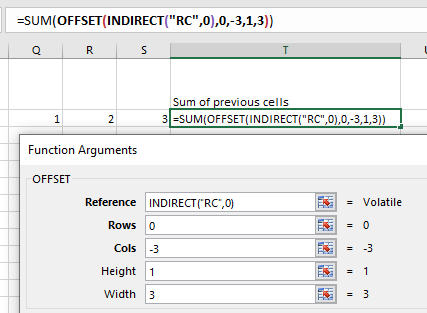The offset can also be specified directly in INDIRECT(“RC[-3]”, 0), in such formulas it can also be useful:

• =ROW() &
• =COLUMN() functions

which, without passing arguments, return the current row and column

### \$ sign for rows

It is not very convenient to specify offsets from the current cell, therefore, for simplicity, a special rule was introduced:

If you specify the current row number with \$ sign, it will be replaced at runtime with actual row number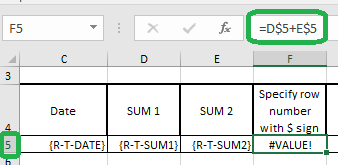The final report will be like this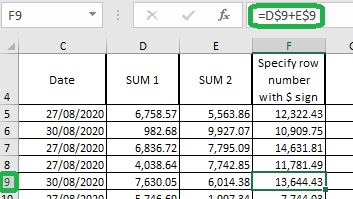PS: For ;direction=column (table output by columns) this rule will accordingly work for formulas in this form \$E

### Shared formulas

Previously, AOK & XTT had “mysterious” formulas disappearing
This bug occurred when a relative link was encountered several times in a row

In the current version (I hope), the conversion from absolute reference to relative and vice versa happens without problems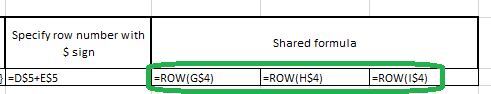### Named cell ranges

By referring to a named range that will be modified at runtime

• The entire range will be stretched once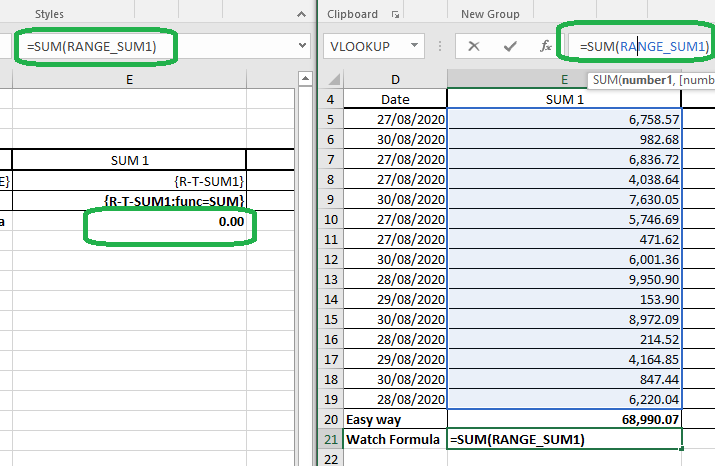• If the name of the range ends with ‘_’ this range will be multiplied and the final list (with all ranges) will be replaced in the formula itself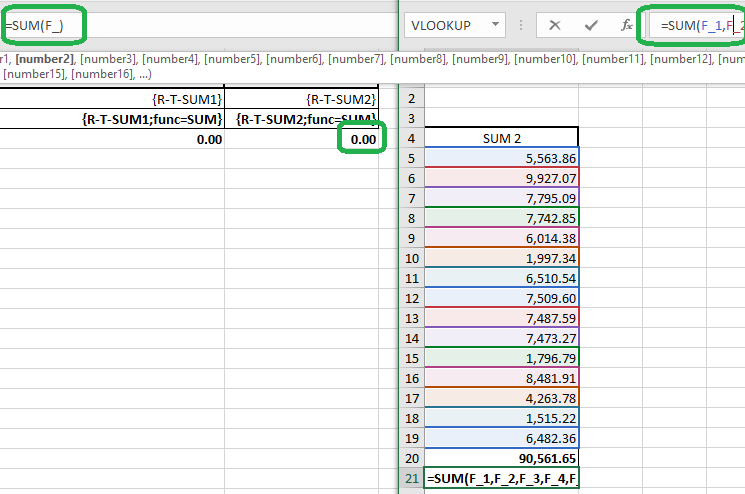### Sum of children

For the sum of children it is not at all necessary to use the previous method with named cells or to create hierarchies

If you need to summarize a table field, you can use standard Excel tools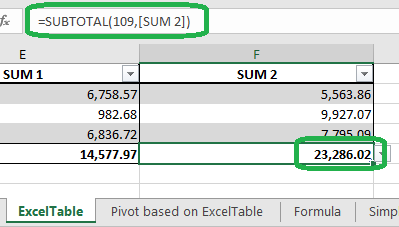### Array formulas

Array formulas are also supported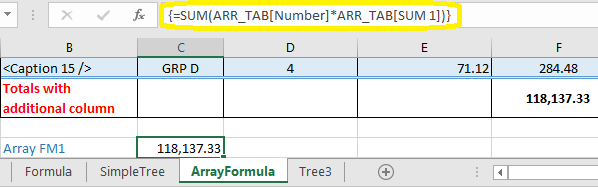### Word & PDF

But what if you need to sum the field values not only in Excel, but also in Word or Pdf? In this case, you can use the aggregation functions ;func= SUM | AVG | COUNT | FIRST

### Conditional formatting formulas

For formulas in conditional formatting, it is advisable to specify the scope as whole columns